# Ciprian Manolescu

What does a topologist do?

I once overheard a conversation between a couple of students in the hallway of a math department. A paper outside the door of each office lists the research interests of the occupant, and one student was surprised: "I'd understand if someone did research in chemistry or engineering, but research in math, that's kind of weird, isn't it?"

It then dawned on me that we mathematicians should do more in terms of explaining what we do to the public. So here is my attempt to explain topology, in a friendly Q & A format.

Q: Why do people do research in math? Isn't math just a bunch of formulas you need to know?

A: Physics, chemistry, biology, economics, computer science, and many other fields use complicated mathematical tools. The job of mathematicians is to develop these tools. In fact, research in pure math is to a large extent about understanding basic mathematical structures. Even if these structures have no practical applications at the moment, they may in the future.

Q: What is topology?

A: The Wikipedia article on topology is quite good, and you can read other people's answers at this site and this site. Let me try to give my own answer.

Topology is the study of geometric shapes, with regard only to those properties that are unchanged by stretching and bending. Geometry deals with properties such as distances and angles, which are of course changed if you stretch or bend the objects. In topology we ignore these kind of properties. From the viewpoint of topology, a square is the same as a circle, since you can deform one into the other! On the other hand, if you have a circle in space, you cannot turn it into a trefoil knot without tearing it. Therefore, the circle and the trefoil are topologically different: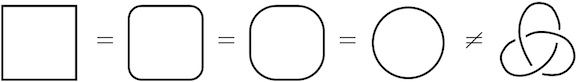One part of topology is knot theory, which classifies knots (closed loops in space). The Knot Atlas is a comprehensive resource about this subject. There is also a YouTube video introducing knot theory.

Although knots live in three-dimensional space, we topologists call them one-dimensional objects, because an ant living on the knot has only one dimension in which to go: along the knot. Similarly, we say that the surface of a sphere is a two-dimensional object, because an ant living on the sphere can go around in two directions.

An amazing topological property of the sphere is that it can be turned inside out, as shown in this video.

Here are a few more two-dimensional shapes, all topologically distinct from the sphere: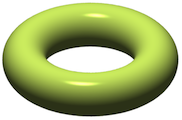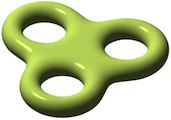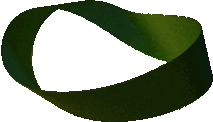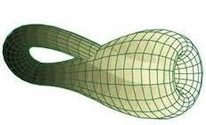These are called the torus, the triple torus, the Möbius band, and the Klein bottle. The last two are interesting because they have only one face. You can order a Klein bottle made of glass at ACME Klein bottles. I have one in my office.

Geometric shapes get even more interesting (and complicated) when you go into higher dimensions. A particularly active area of current research is the study of four dimensional shapes.

Q: Is there a fourth dimension?

A: One can think of time as the fourth dimension, albeit it is a more special one than the other three. There are also theories in physics (such as string theory) that suggest that we live in a universe with 10 or 11 dimensions.

However, it doesn't matter. We topologists study abstract shapes, without regard as to whether they appear in the physical universe. These shapes are still useful as mathematical models for various purposes. For example, if an economist wants to describe the economy in terms of the prices of 28 commodities at any given time, the prices can be viewed as a point in a 28-dimensional space.

It is quite difficult to imagine a 28-dimensional shape. The power of topology is that it gives methods to study such shapes, even if we can't visualize them directly.

Q: What are some famous problems in topology?

A: The Four Color Theorem says that every map can be colored with four colors such that no two neighboring regions have the same color: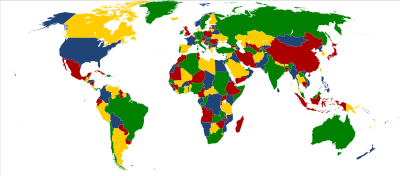This theorem is remarkable because it was the first major result in mathematics that was proved (in 1976) using a computer. The proof is too complex and laborious to be understood by any human without the use of technology.

Another famous problem in topology is the Poincaré conjecture. It says that the three-dimensional sphere is the only smooth, closed, simply connected, three-dimensional shape. (Closed means that the shape is finite and does not have boundary. Simply connected means that every loop in your space can be contracted into a point. In other words, if you throw a lasso onto your shape, you can't really catch anything.)

The Poincaré conjecture took 100 years to be solved. It was finally proved in 2002 by the reclusive Russian mathematician Grigori Perelman. There was a million dollar prize for a solution, but Perelman refused it. You can read more about the story around the conjecture in Donal O'Shea's book.

Q: With the Poincaré conjecture out of the way, what are some remaining open problems in topology?

A: I venture to say that the most important problem not yet solved in topology is the classification of four-dimensional, simply connected, smooth shapes. In principle, one would like to classify all smooth shapes, but there is a theorem that says they cannot be classified. Restricting attention to those that are simply connected makes it a more reasonable problem.

Four-dimensional shapes are more difficult to understand than shapes of any other dimension. Of course, it makes sense that they are more complicated than two or three dimensional objects, but the interesting thing is that they are also more complicated than 47-dimensional objects. Roughly, the reason is that in higher dimensions there is more room to move around, so one can pull apart the parts of our objects that are complicated, and study them separately.

Another interesting problem is to be able to tell if a given knot can be unknotted. Actually, this problem was solved by Haken in 1961, but finding better algorithms is still an active area of research. I worked on this problem myself (with my collaborators Peter Ozsváth and Sucharit Sarkar) and we found a new algorithm in 2006. Here are some slides from a talk I gave describing this work.

Q: Does topology have any applications in real life?

A: Plenty! The kind of shapes studied in topology are used by physicists as models for the universe. Knotting phenomena appear in chemistry and molecular biology, in the study of DNA and other polymers. Fixed point theorems from topology are applied to economics to describe market equilibria. Something called persistent homology is used in the analysis of large data. Simplicial topology has found applications to distributed computing.

To be honest, many of these applications use only "classical" topology, that is, the kind of topology developed 50 or even 100 years ago. Most of the recent results in topology do not yet have any practical applications. However, this is how basic research goes: it comes way before it finds any use in the real world.

There are also those of the opinion that math is more an art than a science, and that discovering new mathematical structures has an intrinsic, aesthetic value. G.H. Hardy, who worked in number theory (another abstract branch of math) in the first half of the twentieth century, was proud to say: "Nothing I have ever done is of the slightest practical use." Nevertheless, nowadays number theory is of utmost importance in cryptography; it is the foundation of internet security. It is quite possible that the topological theories we develop today (such as Floer homology) will never find practical applications, but it is also very possible that they will.

There is also art inspired by topology, such as this fashion show, or the sculptures of Carlo Séquin.